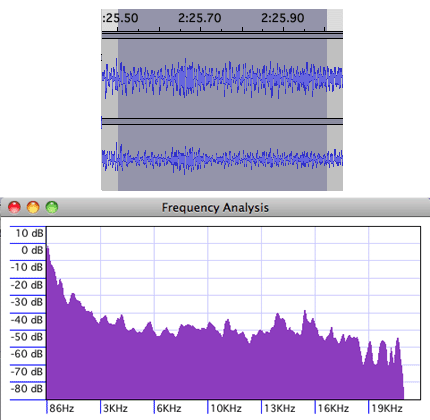# Fast Fourier Transform

Also found in: Medical, Acronyms, Wikipedia.

## fast Fourier transform

[¦fast ‚fu̇r·ē‚ā ′tranz‚fȯrm]
(mathematics)
A Fourier transform employing the Cooley-Tukey algorithm to reduce the number of operations. Abbreviated FFT.
McGraw-Hill Dictionary of Scientific & Technical Terms, 6E, Copyright © 2003 by The McGraw-Hill Companies, Inc.

## Fast Fourier Transform

(algorithm)
(FFT) An algorithm for computing the Fourier transform of a set of discrete data values. Given a finite set of data points, for example a periodic sampling taken from a real-world signal, the FFT expresses the data in terms of its component frequencies. It also solves the essentially identical inverse problem of reconstructing a signal from the frequency data.

The FFT is a mainstay of numerical analysis. Gilbert Strang described it as "the most important algorithm of our generation". The FFT also provides the asymptotically fastest known algorithm for multiplying two polynomials.

Versions of the algorithm (in C and Fortran) can be found on-line from the GAMS server here.

["Numerical Methods and Analysis", Buchanan and Turner].

## FFT

(Fast Fourier Transform) A computer algorithm used in digital signal processing (DSP) to modify, filter and decode digital audio, video and images. FFTs commonly change the time domain into the frequency domain.

FFTs are widely used in voice recognition and myriad other pattern recognition applications. For example, noise-cancelling headphones use FFT to turn unwanted sounds into simple waves so that inverse signals can be generated to cancel them. FFTs are used to sharpen edges and create effects in static images and are widely used to turn a number series into sine waves and graphs.

Fast DFT Processing
The FFT quickly performs a discrete Fourier transform (DFT), which is the practical application of Fourier transforms. Developed by Jean Baptiste Joseph Fourier in the early 19th century, the Fourier equations were invented to transform one complex function into another. One of Fourier's primary goals was to predict the rate of heat transfer based on temperature, mass and proximity. In practice, the terms FFT, DFT and Fourier transform are used synonymously. See DSP.

An FFT TransformA Fourier transform was used to chart the power levels at different frequencies from the half second of digital samples (top).
Copyright © 1981-2019 by The Computer Language Company Inc. All Rights reserved. THIS DEFINITION IS FOR PERSONAL USE ONLY. All other reproduction is strictly prohibited without permission from the publisher.
References in periodicals archive ?
The averaging values reflect the change of wind speed over time of the year and the seasonality of wind environment in the two cities are further investigated in detail by conducting Fast Fourier Transform (FFT) of the one-year wind data and results are shown in Figure 1.
Aggarwal, "Digital image Watermarking using Two Dimensional Discrete Wavelet Transform, Discrete Cosine Transform and Fast Fourier Transform," International Journal of Recent Trends in Engineering, vol.
Zeng, "Measuring residual stress of anisotropic thin film by fast Fourier transform," Optics Express, vol.
The processing procedure are divided into two stages; Fast Fourier Transform (FFT) and Wavelet Analysis (WA).
Finally, all the results via the reduction technique based on complex modes (for the time series, the spectral content, and the limit cycles with the Fast Fourier Transform) are in perfect agreement with the reference performed via the finite element model without any interface condensation.
We combined the matrix form of this discrete Laplace transform with a generalized fast Fourier transform in a purely algebraic fashion, where we used the decomposition of the Laplace transform explicitly and a small number of generalized FFTs as black box.
To design such antenna patterns we adopted an iterative fast Fourier transform method [13, 14].
In this paper, we propose a new fast Fourier transform subspace-based optimization method (NFFT-SOM) which avoids a full SVD of [[??].sub.[partial derivative]], and fast Fourier transform can be used to accelerate the computational speed at the same time.
The purpose of this research is segmentation of optic disc using the combination of Gaussian dan Fast Fourier Transform and median filter as the preprocessing stage and binary thresholding for the detection of the optic disc in the retinal image.
The derived (D), the discrete fast Fourier transform (DFFT), the discrete cosine transform (DCT), and the discrete wavelet transform (DWT).
What this means is a Fast Fourier Transform can decompose A-scans to yield a separate acoustic image for each sinusoid.

Site: Follow: Share:
Open / Close